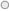# Video - Measure Calculation and the CALCULATE FunctionIntro to CALCULATE()

The first one is the complete and uncut introduction to the CALCULATE function: